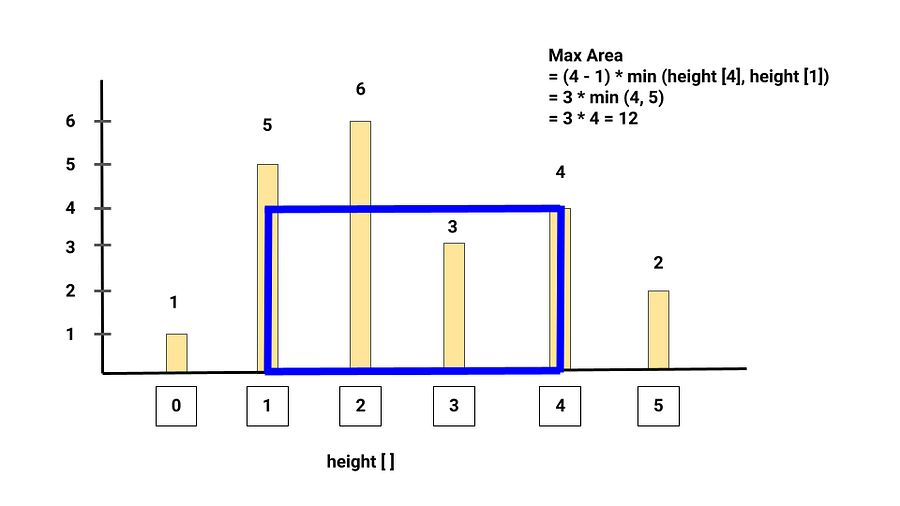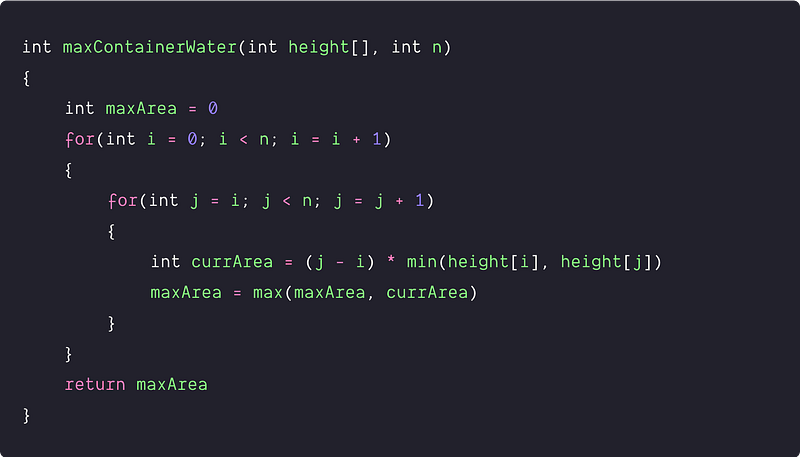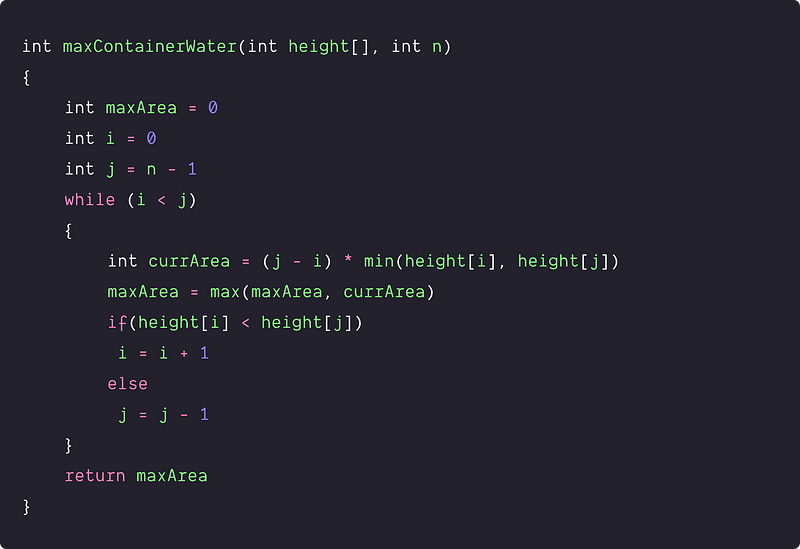# Container With Most Water

Key takeaway: An excellent coding problem to learn problem-solving using the two-pointers approach, where both pointers are moving in the opposite direction. The idea and proof behind the efficient solution are intuitive and worth exploring.

### Let’s understand the problem

Given an array of n non-negative integers height [n], where each value represents a point at coordinate (i, height[i]). Now n vertical lines are drawn such that the two endpoints of line i are at (i, 0) and (i, height[i]). Here each pair of a line with the x-axis forms a container.

Write a program to find two lines, such that the container contains the most water. We should return an integer that corresponds to the maximum area of water that can be contained.

Problem Note

• The value of n is at least 2.
• We need to maximise the area formed between the vertical lines using the shorter line as height and the distance between the lines as the width i.e Area = max [ (j — i) * min (height[i], height[j]) ].

Example

Input: height[] = [1, 5, 6, 3, 4, 2], Output: 12

Explanation: 5 and 4 are distance 3 apart, so the size of the base = 3. Height of container = min(5, 4) = 4, So total area = 3 * 4 = 12. Refer the following image for the better clarity.Important Note: Before moving to the solutions, we recommend learners solve this problem. If solved, then well done! We would like to hear your ideas in the comment. Otherwise no problem, this is an opportunity to learn a new pattern in problem-solving!

### Discussed solution approaches

1. A brute force approach  using nested loops
2. Efficient solution  using two pointers

### A brute force approach  using nested loops

Solution Idea and Steps

The basic idea would be to consider every possible pair of lines and find the maximum area out of those pairs. Area between some pair of lines(i, j) = (j — i) * min (height[j], height[i]) (Think!)

1. Declare a variable maxArea to track the max area between pairs of lines
2. Run two nested loops. Outer loop from i = 0 to n-1 and the inner loop from j = i to n-1
3. For every index i and j of the input, we calculate the area using the above formula and store it in a temporary variable currArea. Now we update the maxArea value i.e maxArea = max (maxArea, currArea)
4. By end of the loop, we return the value stored in the maxArea.

Solution PseudocodeTime and space complexity analysis

We are considering every possible pair of lines using two nested loops. Total no. of pairs = n(n-1)/2 (Think!). Time complexity = O(n²). Space complexity = O(1), we are just using a constant number of variables.

### An efficient solution  using two pointers approach

Solution Idea

Now the critical questions are: How can we improve the efficiency? Is there a possibility to solve this problem in a single scan or O(n) time complexity? What kind of information do we use to optimize it further? Let's think!

In the above approach, we are exploring all the pairs of i and j calculating the area using the formula (j — i) * min (height[j], height[i]). So rather than choosing all pairs, can we cover all possibilities of (i, j) in a wise way and do it using a single loop? Here is an idea!

Case 1: if (height[i] < height[j])

In this case, we move left pointer i to the one right. But why are we doing this? Here is the explanation: When height[i] < height[j], we don’t need to calculate the area for all the pairs between (i, j-1), (i, j-2),…because these areas are smaller than our area at (i, j). How? Let's understand it by comparing the area for (i, j) and (i, j-1).

• A = Area for pair (i, j) = (j — i) * min (height[i], height[j]) = (j — i) * height[i]
• A’ = Area for pair (i, j-1) = (j — 1 — i) * min (height[i], height[j-1])
• if(height[i] < height[j-1]) => A’ = (j — 1 — i) * min (height[i], height[j-1]) = (j — 1 — i) * height[i] < (j — i) * height[i] = A
• if(height[i] > height[j-1]) => A’ = (j — 1 — i) * min (height[i], height[j-1]) = (j — 1 — i) * height[j-1] < (j — i) * height[i] = A'

So overall, A’< A. When A’<A, then all the area between the pairs (i, j-2), (i, j-3),…will be automatically less than A. In other words, we don’t need to calculate the area between the pairs (i, j-2), (i, j-3), etc. That’s why we move the left pointer i to one right in the search of a larger area than A.

Case 1: if (height[i] > height[j])

In this case, we will move the right pointer j to the one left. But why are we doing this? When height[i] > height[j], we don’t need to calculate all (i+1, j), (i+2, j),…because these areas are smaller than our area at (i, j). We can use an idea similar to the above approach for the proof. (Think!)

So here is the simple solution idea - we are taking two pointers, one at the beginning and one at the end of the input height[] array, and maintain a variable maxArea to store the maximum area obtained. At every step, we find out the area formed between the values at the two pointers, update the maxArea and move the pointer pointing to the shorter line towards the other end.

Solution Steps

• We initialize the variable maxArea to store the track the maximum area
• We use two pointers i and j initialised at 0 and n-1 respectively.
• Now we run a loop till the left pointer i is less than the right pointer j
• We compute the area implied by these pointers as (j - i)*min(height[i], height[j]) and update the maxArea.
• if (height[i] < height[j]) then, increment i by 1 else, decrement j by 1
• By end of the loop, our max area gets stored in the variable maxArea.

Solution PseudocodeTime and space complexity analysis

At every iteration of the while loop, we are doing one comparison and moving one pointer by 1. In the worst case, we are scanning each array once using the left or right pointer. Time complexity = O(n)(Think!).

Space complexity = O(1), we are using a constant number of extra variables.

Important Note: We recommend learners transform the above pseudocodes into a favorite programming language (C, C++, Java, Python, etc.) and verify all the test cases. Enjoy programming!

### Critical ideas to think!

• Can we solve this problem using another approach?
• For the area for the pair (i, j), why do we require a minimum of height[i] and height[j]?
• Does the above solution work when values are repeated i.e. any two vertical lines have the same height? How we modify the above code to get the correct output? How do we decide to move pointers?
• Visualize the correctness of the two-pointer approach.

### Comparisons of time and space complexities

• Brute force approach : Time Complexity = O(n^2), Space Complexity = O(1)
• Using two pointers: Time Complexity = O(n), Space Complexity = O(1)

### Similar coding questions to practice

• Trapping rain-water
• Triplet with zero-sum
• Check for pair in an array with a given sum
• The intersection of two sorted array
• Whether an array is a subset of another array
• Merge two sorted arrays of the same size
• Maximum j — i such that A[j] > A[i]
• Count the number of possible triangles
• Find four elements that sum to a given value

Enjoy learning, Enjoy coding, Enjoy algorithms!

Subscribe to get well-designed content on data structures and algorithms, machine learning, system design, oops, and mathematics. enjoy learning!

### We Welcome Doubts and Feedback!